# How to Reverse an Array in C#

## How to Reverse an Array in C# with Examples

In this article, I am going to discuss How to Reverse an Array in C# with Examples. Please read our previous article where we discussed Rotate an Array by K Position using Reversal Algorithm in C# with Examples.

##### How to Reverse an Array in C#?

Here, we are going to reverse the given array.
Example:
Input: {1,2,3,4,5}
Output: {5,4,3,2,1}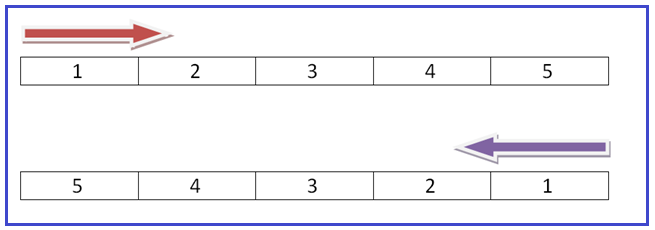Reverse the array horizontally at 90* in place (means not going to use some auxiliary space) we will use the art of swapping.

##### Swapping

Swapping of 2 elements for example 3, and 4 are the two elements of an array and we need to swap these two elements to each other which basically means 3 will be replaced with 4 and 4 will be replaced with 3.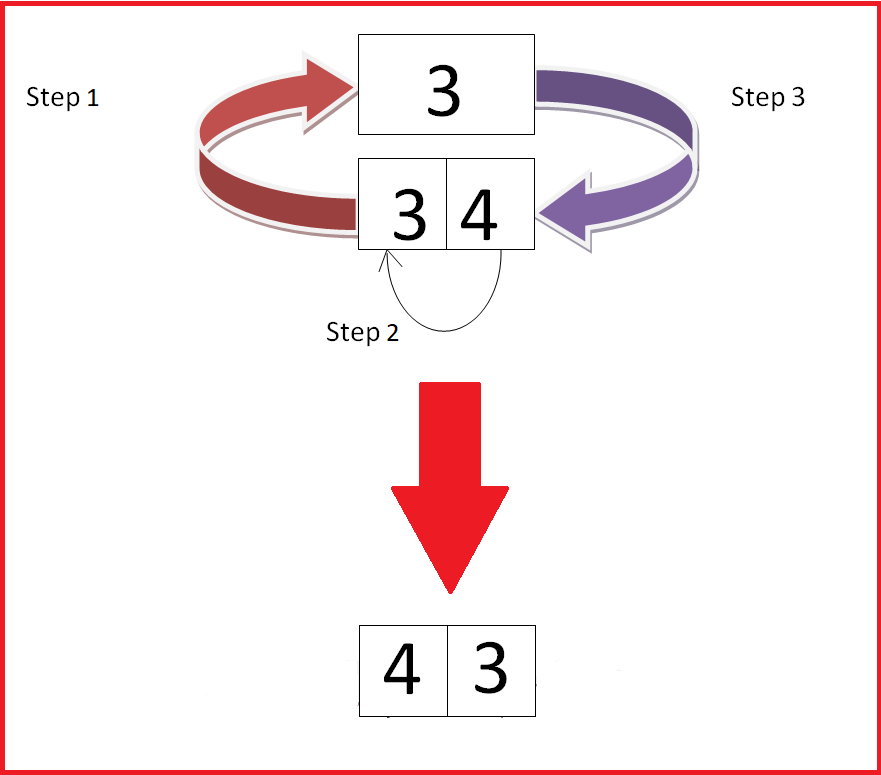To swap elements 3 and 4 to each other.

1. Create a temp variable to store the first index value. (3 will be stored in the temp memory)
2. Replace the second index value with the first index value. (3 will be replaced with 4)
3. Replace the second index value with the temp value. (4 will be replaced with the temp value 3)

To Reverse the Array, we are going to use the swapping technique.

• arr <—-> arr[n-1]
• arr <—-> arr[n-2]
• arr <—-> arr[n-3]

This list will go on until the start index value is less than the end index value.

##### Example to Understand How to Reverse an Array in C#:

Suppose the length of the array is 5. In this case, the swapping of elements will perform as follows:

• arr <—-> arr
• arr <—-> arr
• arr <—->  arr

Graphical Representation of Reversing Array

Step1: 5 and 8 will be swapped with each other.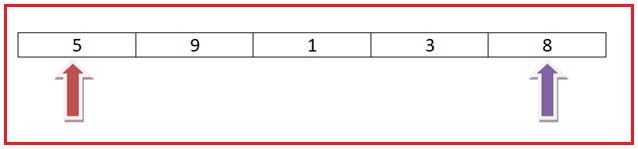Step2: 9 and 3 will be swapped with each other.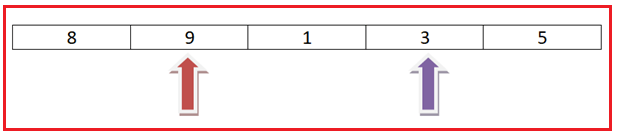Step3: Swapping will stop now as both the start and end index denote the same index value of the array. (startIndex value is not less than endIndex value)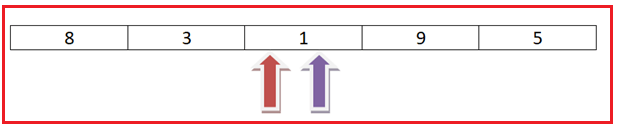##### Algorithm to Reverse an Array:
1. Step1: Create two variables named startIndex and endIndex and initialize values with 0 and (n-1) respectively. (n is the length of the array).
2. Step2: Swap the values of startIndex with endIndex and increment the value of startIndex by 1 (means +1) and decrement the value of endIndex by 1 (means -1).
3. Step3: Repeat Step2 until the startIndex value is less than the endIndex value.
4. Step4: Print the array as a reversed array.
##### C# Program to Reverse an Array
```using System;

namespace ReverseAnArrayDemo
{
public class Program
{
static void Main(string[] args)
{
//Creating an Integer Array
int[] arr = { 1, 2, 3, 4, 5 };

Console.Write("Original Array Elements :");
for (int i = 0; i < arr.Length; i++)
{
Console.Write(arr[i] + " ");
}

ReverseElements(arr, 0, (arr.Length - 1));

Console.Write("\nReversed Array Elements :");
for (int i = 0; i < arr.Length; i++)
{
Console.Write(arr[i] + " ");
}

}

public static void ReverseElements(int[] arr, int startIndex, int endIndex)
{
while (startIndex < endIndex)
{
int temp = arr[startIndex];
arr[startIndex] = arr[endIndex];

arr[endIndex] = temp;

startIndex++;
endIndex--;
}
}
}
}
```
###### Output: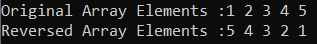Time Complexity: O(n)

Here, in this article, I try to explain Reverse an Array in C# with Examples and I hope you enjoy this Reverse an Array in C# with Examples article.